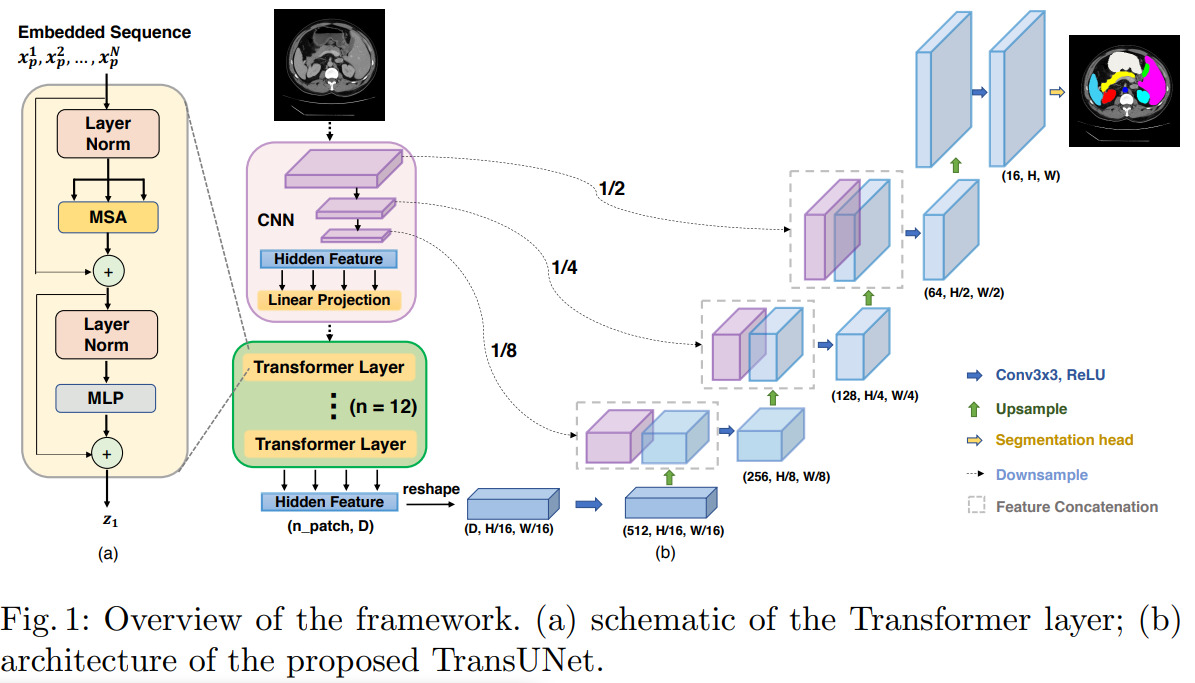# Introduction

In a nutshell, this paper shows how to convert a UNet into a so-called TransUNet by using a visual transformer (ViT) network in the encoder. Details of the architecture are in Figure 1. As opposed to other methods which use a pure transformer-based encoder to convert the input image into a latent vector, the authors use a series of convolutions (much like in the original UNet) to convert the input image into a set of lower-resolution feature maps which they then encode with a ViT.

# Method

The paper starts by summarizing what a ViT is. Starting with an input data $$\mathbf{x}\in R^{H\times W\times C}$$ (which could be the input image or a set of $$C$$ feature maps), $$\mathbf{x}$$ is sliced into $$N$$ non-overlapping patches of size $$P\times P\times C$$ where $$N = \frac{NW}{P^2}$$. The patches are then flatten into 1D vectors and projected into a D-dimensional embedding space with a weight matrix $$E$$ which leads to a set of $$N$$ D-dimensional vectors: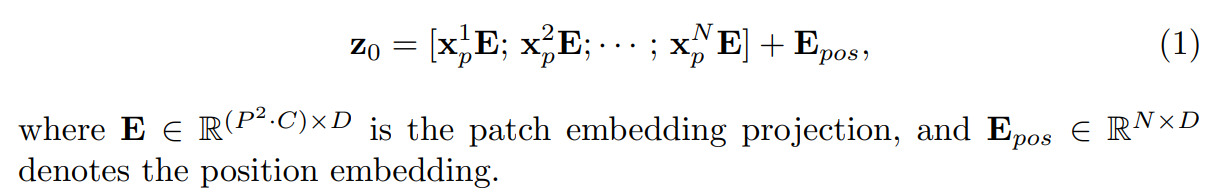The authors mention that by doing so, it allows the system to learn patch embedding positions (hence the use of $$E$$ as weight matrix). The resulting $$z_0$$ embedding vector is fed to the transformer.

As for the transformer, it consists of $$L$$ ($$L=12$$ in their experiments) Multihead Self-Attention (MSA) and Multi-Layer Perceptron (MLP) piped one after the other: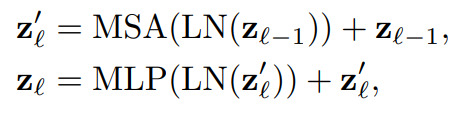where LN is a layer normalization operation. The resulting latent vector (aka hidden feature in Fig.1) is then reshaped and upsampled like a usual UNet.

# Results

They tested their method on two datasets : the synapse multi-organ dataset (30 CT scan images) and ACDC. Results reveal state-of-the-art performances. All experiments were conducted on a single RTX2080 GPU.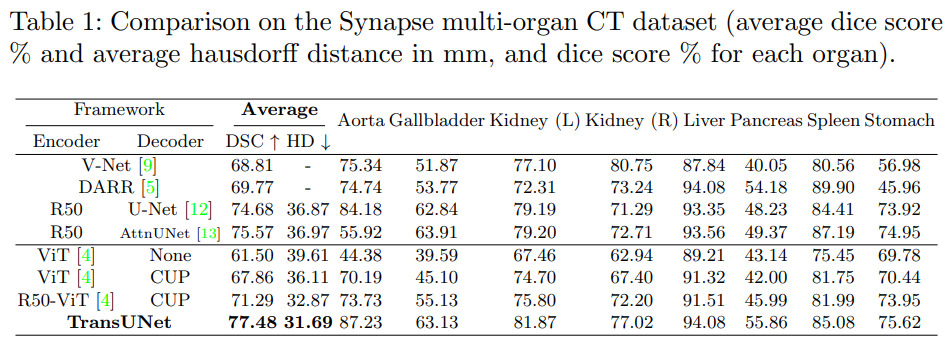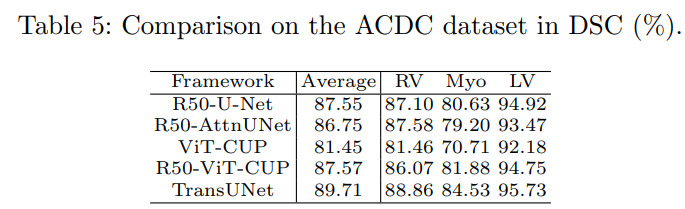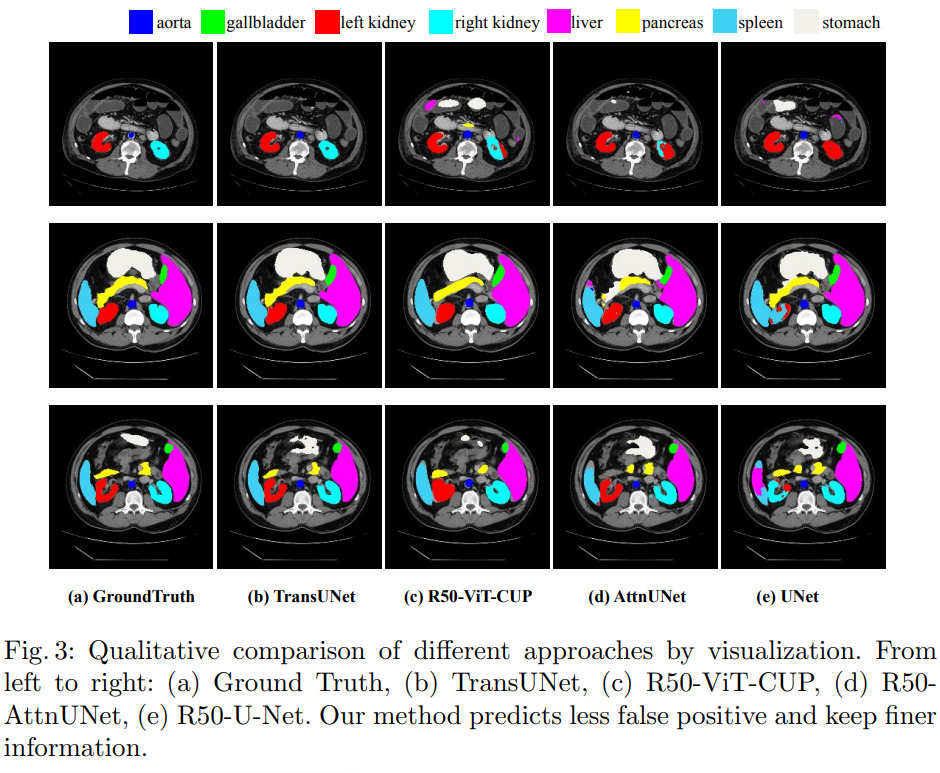CODE: The code is available here : https://github.com/Beckschen/TransUNet